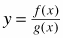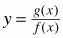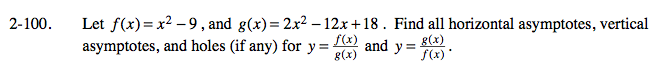### Home > CALC > Chapter 2 > Lesson 2.2.4 > Problem2-100

2-100.

Let f(x) = x2 − 9, and g(x) = 2x2 − 12x + 18. Find all horizontal asymptotes, vertical asymptotes, and holes (if any) forand. Homework Help ✎$y=\frac{f(x)}{g(x)}=\frac{x^{2}-9}{2x^{2}-12x + 18}$

Find horizontal asymptotes by using limits as x → ±∞.

$\lim_{x\rightarrow \infty }\frac{x^{2}-9}{2x^{2}-12x+18}=\frac{1}{2}$

$\lim_{x\rightarrow -\infty }\frac{x^{2}-9}{2x^{2}-12x+18}=\frac{1}{2}$

$\text{Horizontal asymptote at }y=\frac{1}{2}.$

Find vertical asymptotes and holes by factoring and simplifying.

$\frac{x^{2}-9}{2x^{2}-12x+18}=\frac{(x+3)(x-3)}{2(x+3)(x-3)}$

Even though (x − 3) cancels out, there remains an (x − 3) in the denominator. No holes, there is a vertical asymptote at x = 3.

$y=\frac{g(x)}{f(x)}=\frac{2x^{2}-12x+18}{x^{2}-9}$

Find horizontal asymptotes by using limits as x → ±∞.

Find vertical asymptotes and holes by factoring and simplifying.